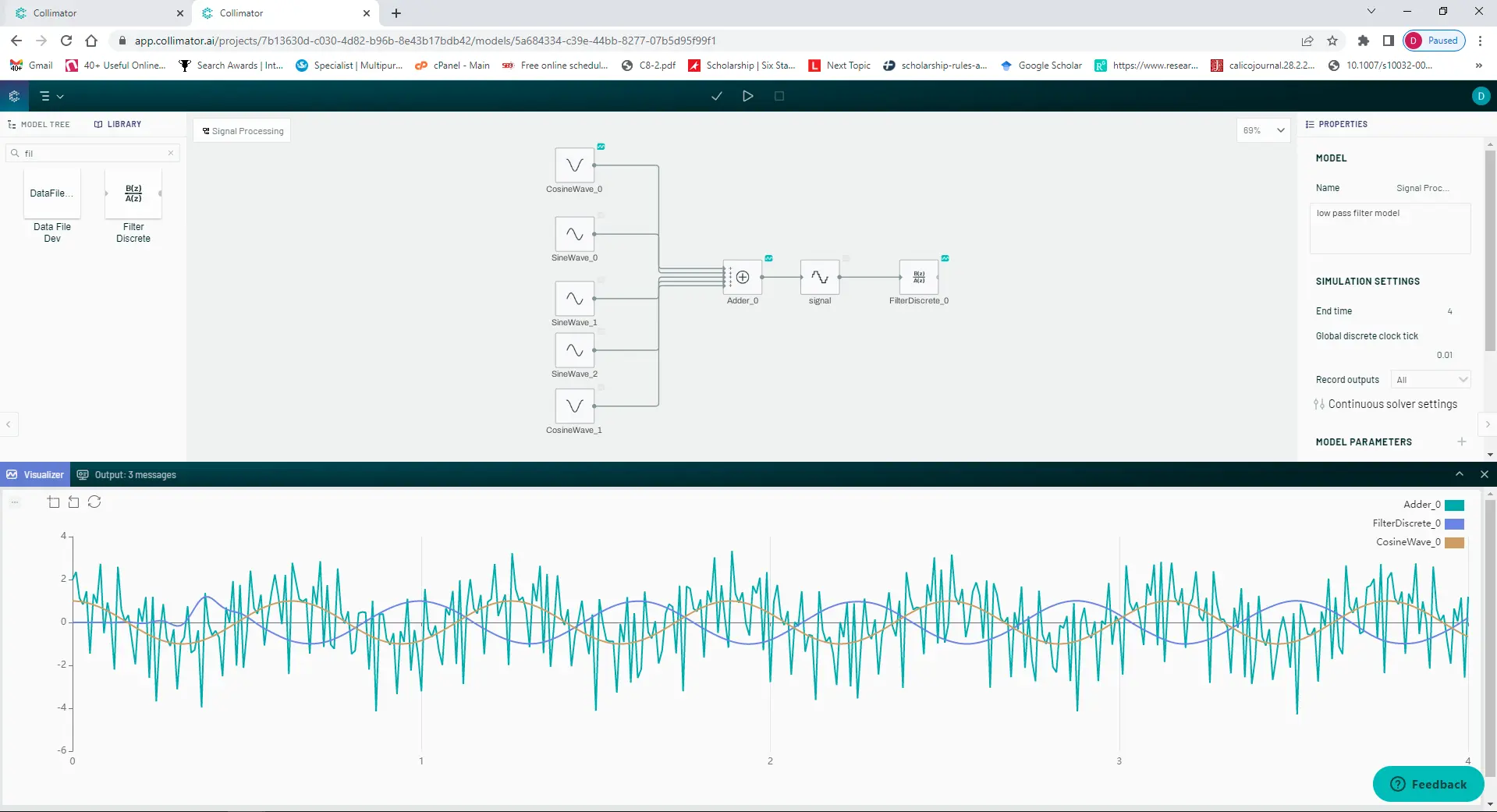# Designing an FIR low pass filterSignal filtering is an integral part of signal processing. In this tutorial we will design a finite impulse response low pass filter that will filter an input signal of our choosing.

## What is an FIR filter?

Finite Impulse Response (FIR) and Infinite Impulse Response (IIR) filters are the two main digital filters used in digital signal processing (DSP). FIR filters differ from IIR filters because they have an impulse response of a finite duration. They are primarily used for tapping of high-orders since IIR filters may become unstable.

## What is a low pass filter?

A low-pass filter is a filter that passes signals with a frequency lower than the selected cutoff frequency. It will attenuate signals with frequencies higher than the cutoff frequency. A low-pass filter is the complement of a high pass filter which lets signals with high frequencies pass.

\begin{array} {|l|l|} \hline Advantages & Disadvantages \\ \hline \text{Always stable} & \text{Require complex computation} \\ \hline \text{Simple to design} & \text{Hard to implement} \\ \hline \text{Easy to optimize} & \text{Can be expensive} \\ \hline \text{Have a linear phase response} & \text{Time consuming to design} \\ \hline  \end{array}

## Modeling our FIR low pass filter in Collimator

We will begin our filter design in Collimator's model editor. First we define the model as shown below:

The model blocks parameters are described in the table below.

\begin{array} {|l|l|} \hline \text{Block Name} & \text{Block Type} & \text{Block Units} & \text{Block Description} \\ \hline \text{PureSignal} & \text{Sine wave block} & \text{Frequency: }10& \text{The target signal} \\ \hline \text{Noise} & \text{Sine wave block}& \text{Frequency: }200& \text{Noise} \\ \hline \text{Noise2} & \text{Sine wave block}& \text{Frequency: }100& \text{Noise} \\ \hline \text{Noise3} & \text{Sine wave block}& \text{Frequency: }250& \text{Noise} \\ \hline \text{Noise4} & \text{Sine wave block}& \text{Frequency: }300& \text{More Noise} \\ \hline \text{NoisySignal} & \text{Adder block}& \text{n/a} & \text{Combines sgnals} \\ \hline \text{Signal} & \text{Quantizer block} & \text{interval: }0.1& \text{Quantizes the signal } \\ \hline \text{FilteredSignal} & \text{Filter discrete block}& \text{Filter type: FIR}& \text{Incorrect B coefficients} \\ \hline  \end{array}

In order to ensure our model works we must specify our end time which will correspond to the duration of our signals and our global discrete clock tick whose inverse will correspond to our signal's sample rate.

## Solving for FIR low pass filter coefficients in Collimator

In order to solve for the correct filter coefficients to apply to our filter block we will need to use a Collimator notebook. Using Scipy, Numpy and Matplotlib, we will run calculations to solve for the coefficients.

We begin by importing the libraries we will use:

import collimator as C
import numpy as np
import scipy as sp
import pylab as pb



Next we call our model and run it in order to obtain the noisy input signal and assign it to a numpy array

# retrieving block parameters and calculating the sample rate and number of samples
sample_rate = 1/signal_model.configuration.discrete_step
nsamples = signal_model.configuration.stop_time*sample_rate

sound = C.run_simulation(signal_model).results
time = sound['time'].to_numpy()
sinewave = sound['signal'].to_numpy().reshape(len(time)) # ensure this is reshaped into 1D array



Now we create a visualization of the frequency response of our noisy input signal

# visualizing the frequency response of our noisy signal
w,h = sp.signal.freqz(sinewave)
pb.plot(w*sample_rate, abs(h))



Now we begin designing our filter. The cutoff frequency of our filter will be 10Hz. As a result the output signal should be the same as our original source signal.

# designing the filter
# defining the nyquist rate
nyq_rate = sample_rate / 2.0
# defining the width od the filter from pass to stop (relative to the nyquist rate)
# set it at 5 hz
width = 5/nyq_rate
# setting the attenuation in the stop band
ripple_db = 60
# computing the order and Kaiser parameter for the FIR filter
N,beta = sp.signal.kaiserord(ripple_db,width)
# defining our cuttoff frequency
cutoff_hz = 10.0
# using firwin with a kaiser windo to define our coefficients for the filter
taps = sp.signal.firwin(N, cutoff_hz/nyq_rate, window = ('kaiser',beta), pass_zero='lowpass')
# using lfilter we filter our noisy signal
filtered_signal = sp.signal.lfilter(taps,1.0,sinewave)



Now we plot the filter's frequency response in order to ensure it is a low pass filter

# viewing our filters response signal
pb.figure(2)
pb.clf()
w,h = sp.signal.freqz(taps, worN = 8000)
pb.plot((w/np.pi)*nyq_rate,np.absolute(h),linewidth=2)
pb.grid(True)
#filter has a width of around 5 hertz as specified


# view our taps for our model.